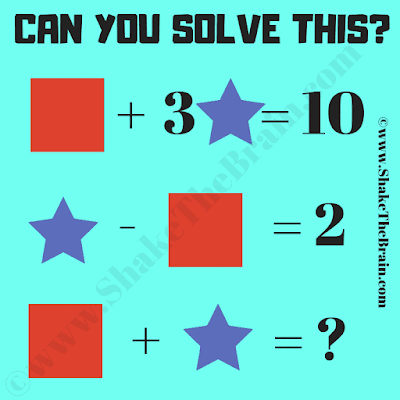Here are some Maths Shapes for which combined weight is given in different Maths equations. Can you solve these Maths equations to find the weight of each of these shapes and then solve the last equation to find the value of the missing number?Can you find the value of the missing number?
Answer of this "Maths Shapes Equations Picture Puzzle", can be viewed by clicking on the answer button.

#### 1 comment:

Unknown said...

There are two answers. The one you have here but also the answer could be 6 with Star = 2 and Square = 4.